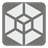# Geometry Figures

Simple
English RU

You often work with the different geometrical figures and with their parameters - the perimeter, area, and volume. You are tired of doing it manually, so you’ve decided to automate this process, and therefore you need to write a particular program. In this program you should create the class Parameters which will choose one of the few figures (the circle, regular triangle, square, regular pentagon, regular hexagon, cube) using the choose_figure() method and will count the perimeter, area, and volume with the help of the following methods:

perimeter() - returns the perimeter of the figure;
area() - returns the area of the figure;
volume() - returns the volume of the figure.

Also you have to create a class for each figure and use the Strategy design pattern to solve this mission.
Every figure, except the cube, has the volume = 0.
If the result has no decimal places, you should return it as int(), in other case - round it to the 2 decimal points.

Examples:

```figure = Parameters(10)

figure.choose_figure(Circle())
figure.area() == 314.16

figure.choose_figure(Triangle())
figure.perimeter() == 30

figure.choose_figure(Square())
figure.area() == 100

figure.choose_figure(Pentagon())
figure.perimeter() == 50

figure.choose_figure(Hexagon())
figure.perimeter() == 60

figure.choose_figure(Cube())
figure.volume() == 1000
```

Input: Statements and expressions of the classes.

Output: The perimeter, area, and volume (number).

Hot it is used: For the geometrical object analysis.

Precondition: All data is correct.21
Settings
Code:
Other:
Invalid hot key. Each hot key should be unique and valid
Hot keys:
•  to Run Code: to Check Solution: to Stop:
CheckiO Extensions

CheckiO Extensions allow you to use local files to solve missions. More info in a blog post.

In order to install CheckiO client you'll need installed Python (version at least 3.8)

Install CheckiO Client first:

`pip3 install checkio_client`

`checkio --domain=py config --key=`

Sync solutions into your local folder

`checkio sync`

(in beta testing) Launch local server so your browser can use it and sync solution between local file end extension on the fly. (doesn't work for safari)

`checkio serv -d`

Alternatevly, you can install Chrome extension or FF addon

`checkio install-plugin`
`checkio install-plugin --ff`
`checkio install-plugin --chromium`

Read more here about other functionality that the checkio client provides. Feel free to submit an issue in case of any difficulties.